# Percentages Lesson Plan: Teaching Students to Comprehend and Solve Word Problems Using Percentages

## Lesson Objective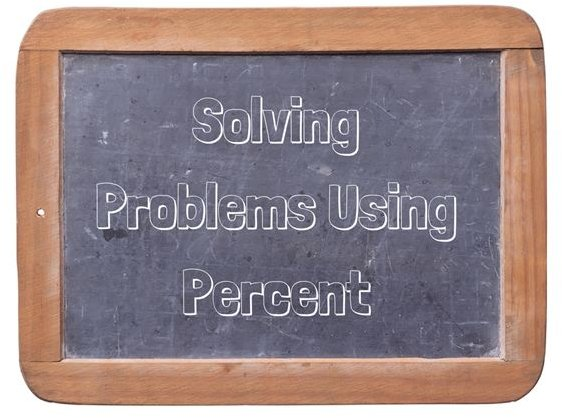The lesson is aligned to the Common Core State Standards for Mathematics – 6.RP.3.c Ratios and Proportional Relationships – Find a percent of a quantity as a rate per 100; solve problems involving finding the whole, given a part and the percent.

## Lesson Procedure

Find a percent of a quantity as a rate per 100.

There are 4 red cars in the parking lot.

There are a total of 20 cars in the parking lot.

• A ratio can be written to show how the number of red cars relates to the total number of cars. The ratio can be written as 4 to 20 or 4 : 20 or 4/20.
• A percent can be written to show how many cars are red for every 100 cars.

Complete the table to show the percent of red cars in the parking lot.

What percent of the cars in the parking lot are red?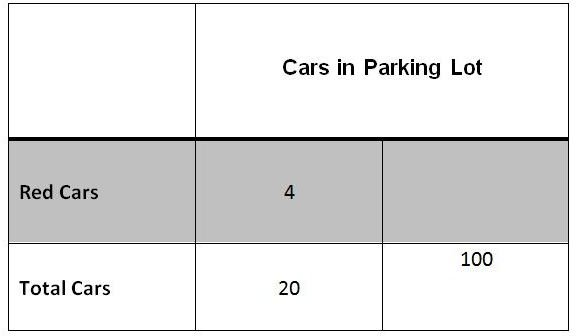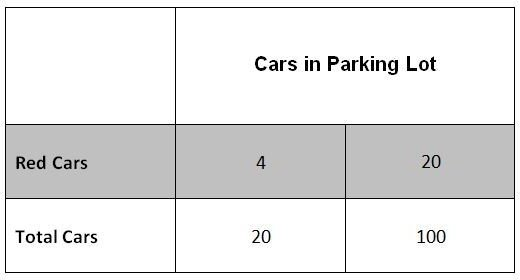Multiply 20 x 5 = 100, so multiply 4 x 5 = 20.

20% of the cars in the parking lot are red.

## Individual or Group Work

Solve problems involving finding the whole when given a part and the percent.

Janet took a test in science and correctly answered 19 of the 25 questions.

Janet took a test in spelling and correctly answered 18 of the 20 questions.

1. Complete the table.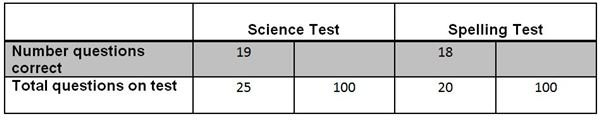2. On which test (science or spelling) did she do better?

Janet took a test in history and correctly answered 21 of the questions and received a score of 84%.

Janet took a test in mathematics. There were a total of 10 questions on the test and received a score of 90%.

3. Complete the table.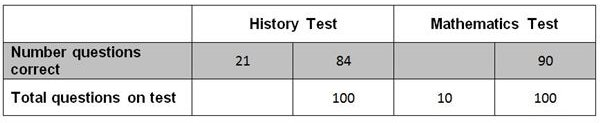4. How many total questions were on the history test?
5. How many questions did Janet correctly answer on the mathematics test?
6. The ratio of correct answers to the total questions on the test is 85 to 100. There were a total of 20 questions on the test. How many questions were answered correctly?

Solve problems using percent.

A school library has 16,250 books.

7. 40% of the books in the school library are realistic fiction books. How many books are realistic fiction?
8. 20% of the books in the school library are science fiction books. How many books are science fiction?
9. 30% of the books in the school library are reference books. How many books are reference books?
10. 10% of the books in the school library are biographies. How many books are biographies?

1. Complete the table.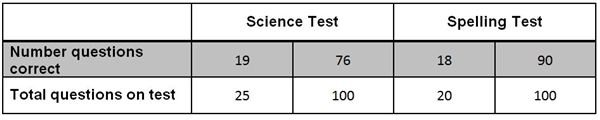2. Spelling Test
3. Complete the table.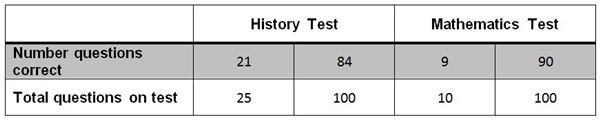4. 25 questions
5. 9 questions
6. 17 questions were answered correctly
7. 6,500 realistic fiction books
8. 3,250 science fiction books
9. 4,875 reference books
10. 1,625 biographies

## Concluding Statement

Students should be able to solve problems finding the whole, given a part and the percent.

## This post is part of the series: Ratios, Rates and Percentages

This series of lesson plans provides you with classroom teaching tool ideas as well as group or individual work example problems and answers you can use to help your students comprehend ratios, rates and percentages. The lessons align to the Common Core State Standards for Mathematics.